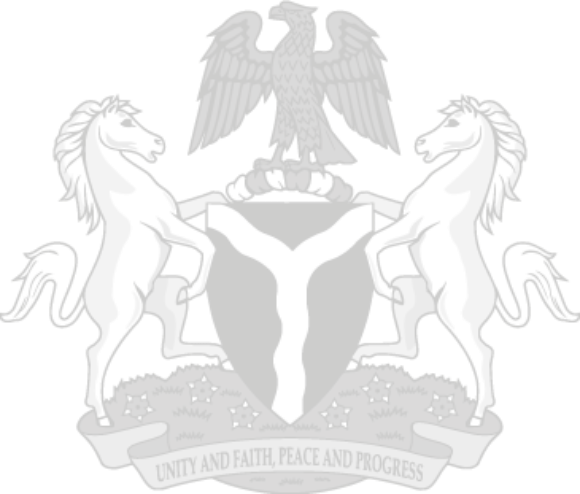## Foreign Trade in Goods Statistics Q1 2018

Total Imports value was N2, 518.26 billion in Q1, 2018, 19.22% higher than Q4, 2017 (N2,112.31 billion) and
8.04% higher than Q1, 2017 (N2,330.91 billion).

The value of imported agricultural goods in the first quarter of 2018 (N184.49 billion)
declined by 18.90% from Q4, 2017 (N227.49 billion) and by 5.87% from Q1, 2017 (N196.0 billion).
- Raw materials imports in Q1 2018 (N284.81 billion) grew by 1.93% compared to Q4, 2017
(N279.41 billion) and by 9.89% compared to Q1, 2017 (N259.17 billion).
- Solid minerals imports in Q1, 2018 (N12.62 billion) decreased by 17.27% on a quarter-to-quarter
basis (N15.26 billion), however increased by 3.67% on a year-to-year basis (N12.17 billion).
- Energy goods imports in Q1, 2018 (N32.4 million) recorded 76.51% lower than Q4 2017 (N138.1
million), and 205.28% higher than Q1, 2017 (N10.6 million).
- The value of manufactured goods imported in Q1 2018 (N1,189.97 billion) declined by 1.65% over
the previous quarter (N1,209.96 billion) but increased by 12.11% over the same quarter in 2017
(N1,061.42 billion).
- The value of Other oil products imported in Q1,2018 (N846.31 billion) was 122.70% higher than
then value in Q4, 2017 (N380.03 billion) and 5.51% higher than Q1, 2017 (N802.11 billion).

Total export value amounted to ? 4,693.34 billion in Q1, 2018, representing a significant growth of 20.02%
over Q4, 2017 (N3,910.62 billion) and 56.01% over Q1, 2017 (N3,008.33 billion).

The export value for Agricultural goods in Q1, 2018 (N73.24 billion) was 63.84% higher than the
value in Q4, 2017 (N44.70 billion) and 24.01% higher than the value recorded in Q1, 2017 (N59.06
billion).
- Raw material exports value decreased by 13.62% in Q1, 2018 (N32.70 billion) compared to Q4, 2017
(N37.85 billion) but increased by 47.71% compared to Q1, 2017 (N22.13 billion).
- Solid Minerals exports value in Q1 2018 (N26.92 billion) recorded a 12.96% quarter on quarter
growth (N23.83 billion), and a 58.50% year on year growth rate compared to Q1, 2017 (N16.98
billion).
- The value of Energy goods exported in Q1, 2018 (N10.39 billion) was 8.8% higher than that of Q4,
2017 (N9.55 billion) and 16.5% higher than the value in Q1, 2017 (N8.92 billion).
- Manufactured goods exports in Q1 2018 (N434.37 billion) saw significant growth by 684.11% over
the previous quarter (N55.39 billion), and by 576.90% when compared to Q1, 2017 (N64.17 billion).
- The value of Crude Oil exports recorded in Q1, 2018 (N3,580.01 billion) was 10% higher than the
value in Q4, 2017 (N3,251.61 billion) and 50.74% higher than the value in Q1, 2017 (N2,374.94
billion).
- Other oil products exports in Q1, 2018 (N535.8 billion) also grew steadily in the reviewing quarter,
with a 10.53% growth from Q4, 2017 (N484.65 billion) and a 15.92% growth from the value recorded
in Q1, 2017 (N462.10 billion).

Data source: National Bureau of Statistics

### INFOGRAPHICS### Portal & Apps